### Journal of Model Based Research

Current Issue Volume No: 1 Issue No: 3

Research Article Open Access Available online freely Peer Reviewed Citation

# Genetic Algorithm Coupled with Neural Networks to Guesstimate the Subsurface Features of the Earth

### A. Stanley Raj 1,   Y. Srinivas 2,   R. Damodharan 2,   B. Chendhoor 2,   M. Sanjay Vimal 2

1Department of Physics, Loyola College, Chennai

2Centre for GeoTechnology, Manonmaniam Sundaranar University, Tirunelveli, Tamil Nadu-627012, India.

## Abstract

Electrical resistivity method is often used to estimate the subsurface structure of the earth. Many inversion algorithms are available to estimate the subsurface features. However, predicting the exact parameter in the non-linear subsurface of the earth is difficult because of its complex composition. Soft computing tools can approximate the subsurface parameters more clearly. Each soft computing tool has certain advantages and disadvantages. A hybrid formation of algorithms will make the decision more appropriate than depending on a single tool. Here in our study the data obtained through Vertical Electrical Sounding has been used to determine the sub surface characteristics of earth viz., true resistivity and thickness. Artificial Neural Networks (ANN) requires certain optimizing procedures. Here in this paper, Genetic Algorithm (GA) is applied to optimize Artificial Neural Networks (ANN). This coupled approach is tested with the field data. Error percentage of algorithm nearly mimics the behavior of earth and is verified. The best performance result shows that this technique can be implemented to estimate the non-linear characteristics of the earth more noticeably.

##### Author Contributions
Received 22 Jun 2020; Accepted 23 Jul 2020; Published 24 Jul 2020;

Academic Editor: Masoud Vakili, Iran University Science and Technology, Iran.

Checked for plagiarism: Yes

Review by: Single-blindThis is an open-access article distributed under the terms of the Creative Commons Attribution License, which permits unrestricted use, distribution, and reproduction in any medium, provided the original author and source are credited.

Competing interests

The authors have declared that no competing interests exist.

Citation:

A. Stanley Raj, Y. Srinivas, R. Damodharan, B. Chendhoor, M. Sanjay Vimal (2020) Genetic Algorithm Coupled with Neural Networks to Guesstimate the Subsurface Features of the Earth. Journal of Model Based Research - 1(3):13-27. https://doi.org/10.14302/issn.2643-2811.jmbr-20-3449

## Introduction

In recent times, people moved towards advanced techniques to model the complex behavior system associated with nonlinearity, high-order dynamics, time-varying behavior, and imprecise measurements.

One of such non-linear characteristic behavior that we know is the field of Earth Sciences. Because of the complex structural behavior of rocks and soil formations, it is difficult to fix each parameter in a certain range. So the efficient soft computing tool is needed to estimate the subsurface parameters of the earth that are pertinent to the real world.

Geophysical inverse problems atmost are nonlinear in nature and pocessess irregular objective function which is of multimodel type 1. The traditional approaches over such geophysical problems can leads to failure due to the initial model that are closer to some local minimum which will attract it. As these problems pocess the objective function that shows several local maxima and minima, they can be optimized through sophisticated approach by global optimization techniques. Evolutionary Algorithms which are generally known as global optimization technique, are appropriate for providing solutions to the irregular nonlinear problems of geophysics. 1, 2, 3 have successfully been implemented this technique to solve many types of problems. The possible nature of this technique paves ways to locate the global optimum with the actual presence of many local optima, they are having major advantage to evolutionary computation is that it can easily gets parallelized to run on multiple modes of processors simultaneously. In a single generation as many as several numerous hundred objective function evaluations can be made, but each of them has been independently as a results of any other, so the process of parallelizing of the algorithm can theoretically increase efficiency by the means of orders of magnitude.

In recent years, Genetic Algorithm plays a significant role in optimizing various geophysical problems which includes Seismic waveform inversion , Marine Electromagnetic data inversion , Inversion of data obtained through surface waves and that have been proved by many researchers. 4, 5, 6, 7, 8, 9

Here in our study Artificial Neural Networks (ANN) and Genetic Algorithm (GA) were combined to perform a best unification algorithm for predicting the subsurface features of the earth. Neural networks can approximate the result based on the training. It can exhibit mapping capabilities easily between the input and output patterns. Here synthetic apparent resistivity data were trained with ANN algorithm and tested with the field data. As ANN can learn by examples, the training dataset was developed synthetically and tested. The layer model provides the information about the true resistivity and the thickness of the subsurface layer with error percentage.

Genetic algorithm (GA) is a search algorithm based on the mechanics of natural selection and natural genetics. The central theme of research on genetic algorithms has been robustness, the balance between efficiency and efficacy necessary for survival in many different environments. It is computationally powerful tool that seeks to reproduce mathematically the mechanics of natural selection and natural genetics, according to the biological processes of survival and adaption 10. GAs excels in solving complex nonlinear problems by making use of enormous parallel information to search more efficiently. As they are highly capable of finding the global optimum with the presence of local optima, discontinuities and noises , so this stands as a major advantage for optimizing geophysical problems rather than other techniques.

Many applications were done on the recent years based on artificial intelligent techniques tocontrol, prediction and inference studies 11, 12, 13, 14, 15. The fuzzy modeling was first explored by Takagi and Sugeno 14 and later ANFIS network was developed by Jang in 1993 16. An adaptive network is a multilayer feed forward network in which each node performs a particular function on giving the input to the network. Electrical resistivity data collected from the VES (Vertical Electrical Sounding) data is used for training the data set. Trained data set will be the reference data for interpreting the sub surface layer parameters of the earth. This dataset will be used to train ANN that best model this data. Layer parameters viz., apparent resistivity and Thickness of earth’s sub surface can be estimated using this tool with less error percentage.

Genetic Algorithm coupled Neural Networks (GA-ANN)approaches the combined behavior of genetically optimized neural network system. Input data are categorizing to seek the objective function using genetic algorithm which is one of the best global search optimization technique can able to find the global optimum minimum. They can be used to modeling or control linear or non-linear systems using ANN. ANN can generate the synthetic data required for geoelectrical inversion. GA searches the best-fit optimization generated the synthetic data and interpretation was done by the ANN model. The best-fitting individual of the population in GA will predict the output with less error percentage

Our Study area comprises of Rajapalayam-Alangulam Belt of Virudhunagar District , Tamil Nadu State in Indian subcontinent. This area lies in the southern part of the state in the Eastern slopes of Western Ghats. The Study area is endowed with various mineral and water resources. To study the layered structure of the Earth with the delineation of aquifer parameters , the major problem that has to be optimized in this area is the mixed and complex nature of geological formation in that area . Vertical Electrical Sounding method provides a good solution for that. A wide variety of optimization techniques have been used to interpret Vertical Electrical Sounding(VES) data of geophysical prospecting but this coupled approach of GA-ANN shows better results in obtaining information regarding layered structure of the Earth

## Geophysical Method

Geophysical exploration techniques are vibrant and powerful tools that play a vital role in the delineation of aquifer parameters in different geological formations. In particular, The Geophysical method consisting of vertical electrical sounding (VES) survey has been proved to know the variation of resistivity of the aquifer parameters 17. Schlumberger electrode array having principle advantage over several types of arrays , (Figure 1) is used to study the electrical resistivity distribution of the subsurface in order to understand the groundwater conditions. The Vertical Electrical Sounding provides a non-destructive,fast and economic way to study properties of aquifers. An important advantage of VES method is that quantitative modelling is possible using either model curves or software. The resulting models provides accurate estimates of electrical resistivity, thickness and depth of subsurface strata of the Earth. This array is a powerful tool in delineation of groundwater potentials because of its simplicity nature and cost effective. The field procedure involves, among the four electrodes that are used, the potential electrodes (M and N) remain fixed and the current electrodes (A and B) are expanded symmetrically about the centre of the spread (Figure 1). With very large values of current electrodes, however, it is necessary to increase the potential electrodes. Maximum half current electrodes (AB/2) separation used in this survey is 100 meters. Usually the depth of penetration is proportional to the separation between the electrodes and varying the electrode separation provides information about the stratification of the ground.

Figure 1.Schlumberger Electrode Configuration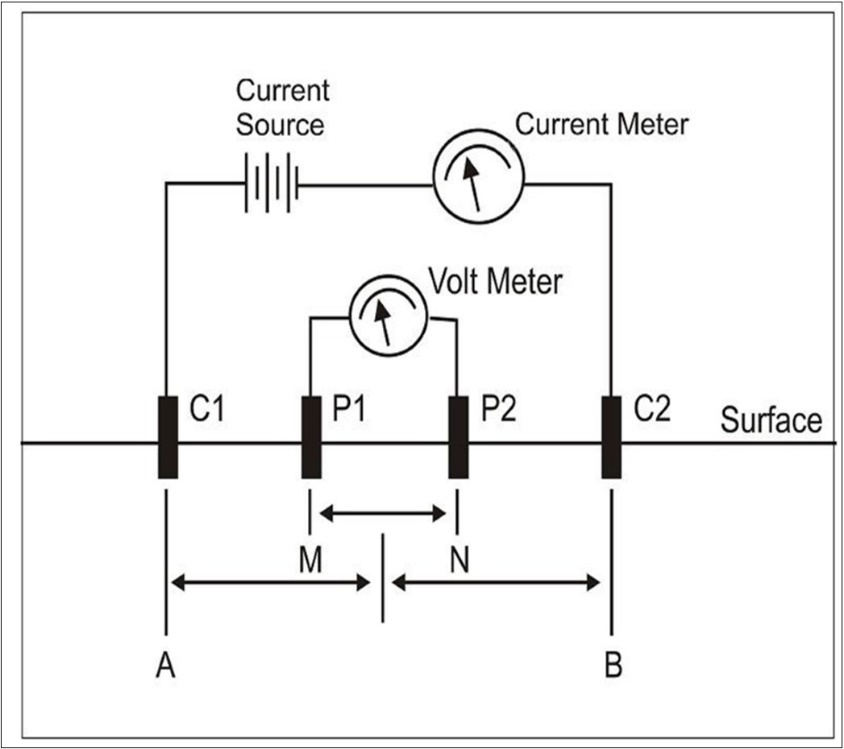## Artificial Neural Network Architecture

Artificial neural network can play a major role to dig out the mystery of earth science by means of the previous learned examples. These networks have self-learning capability and are fault-tolerant as well as noise-immune and have applications in various fields 18.

A very important feature of these networks is their adaptive nature, whose ‘learning by example’ replaces “programming” in solving problems. This feature makes such computational models very appealing in application domains where one has little or incomplete understanding of the problem to be solved but where training data is readily available. ANNs are now being increasingly recognized in the area of classification and prediction, where regression model and other related statistical techniques have traditionally been employed. Introducing the layer of neurons involved in the training is shown in (Figure 2). The most widely used learning algorithm in an ANN is the back propagation algorithm. There are various types of ANNs like multilayered perception, radial basis function and kohonen networks. In fact majority of the network are more closely related to the traditional, mathematical and/or statistical models such as non-parametric pattern classifiers, clustering algorithms, non-linear filters, and statistical regression models. ANNs have been wed for a wide variety of applications in the field of non-linearity.

Figure 2.Input element vector of ANN with layer of neurons having certain weights and biases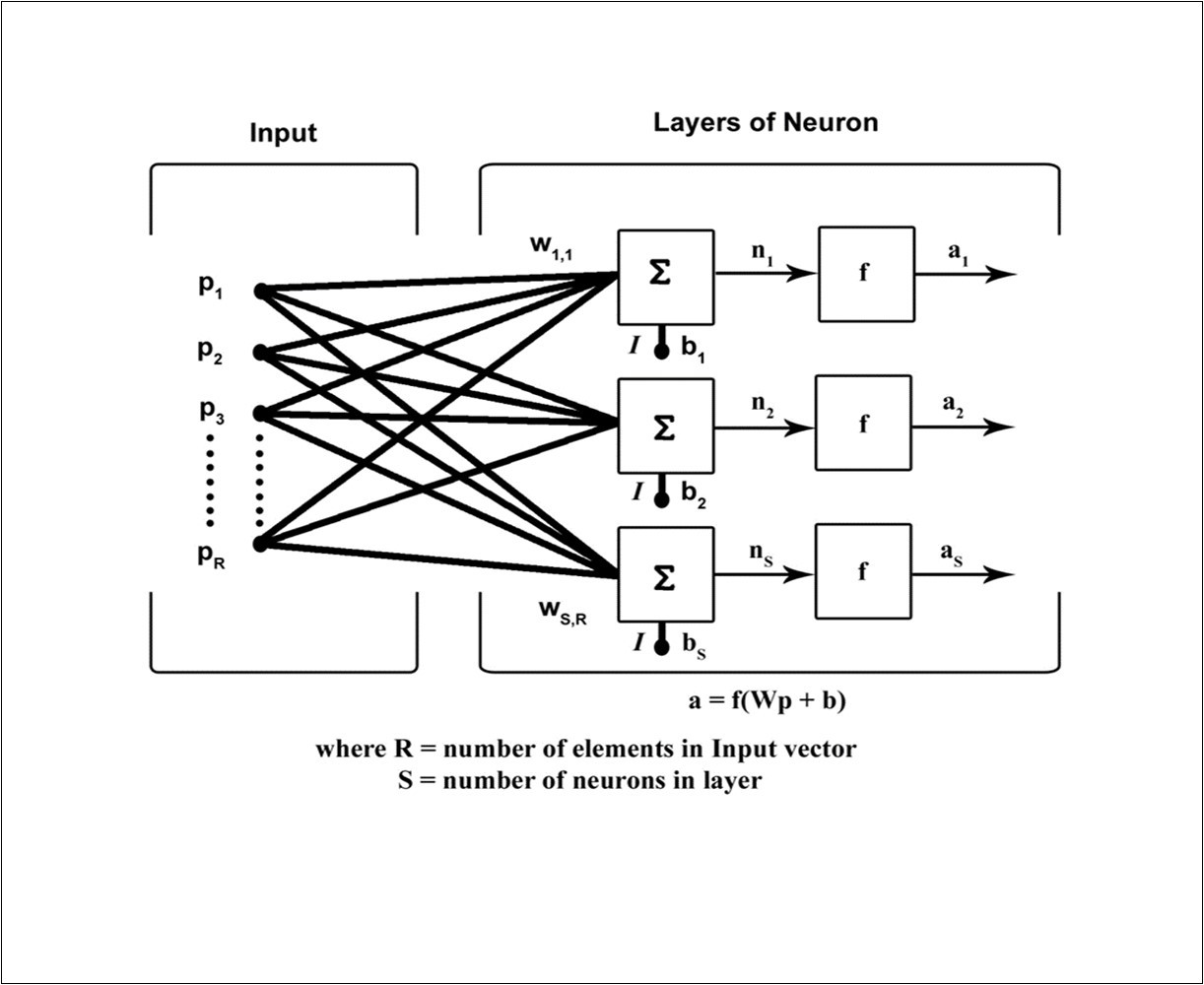Here the sigmoid function is used as activation function. It can produce outputs with reasonable discriminating power and its output functions are differentiable, which is essential for the back propagation of errors 19.During FNN training, each hidden and output neuron process inputs by multiplying each input by its weights. The products are summed and processed using the activation function. The expression for logistic sigmoid function and its derivative is given below.

f(x)= 1/1-e-x

F1(x) = f(x)(1-f(x))

The number of neurons in the hidden layer varies as per the requirement of optimum performance, which could be decided on trial and error basis. Initial weights are assigned at random in the range suitable for the activation function at neurons 20.

In this paper an iterative non-linear feed forward network backpropagation algorithm and the performance was tested.

## Genetic Algorithm

Various optimization problems that can be solved by the method of Genetic Algorithm (GA), which is based on natural selection particularly of biological evolution. GA is generally mimicking the process of natural evolution, which generates useful solutions for optimization problems using techniques inspired by natural evolution, such as inheritance, mutation, selection and crossover 10. The genetic algorithms are inspired by Darwin's theory of evolution that

To ensure their survival, the respective individual Organism must be over reproductive.

1. In the next generation, some minor stochastic changes are seen.

2. The more successful will be better adaptive individuals which will be able to pass their genes to the successive generations.

To optimize a particular problem GA has five major steps, that are as follows;

A random initial population of the problem that has to be optimized is generated by the means of random generator. Initial population is represented by the set of parameter values which describes the problem. These set of parameters are then transformed into a string of binary bits (0’s and 1’s) of specific length. At each and every generations, the chromosomes are tested for fitness using fitness function which is achieved by decoding binary strings into set of parameter values

By the means of various ranking schemes which results that good chromosomes have higher chances to be in the next generation. Rank selection schemes performs the operation over the population on the basis of fitness values. In particular over various ranking schemes tournament selection is preferable 10. In tournament selection process, a set of random individuals is selected from the existing generation and the individuals which processes high fitness values are forwarded to next generation.

The process of cross over and mutation is performed over existing generation to create new generation which is based on crossover and mutation probability.

The new generation is formed by replacing the currently existing generation. The children of the successive generations are decided based upon the fitness value through fitness function provided.

The process of GA stops when at least one among the various stopping criteria such as Maximum number of generations, Maximum time limit, Maximum fitness limit etc., met.

Conventional search methods are not robust. The robustness behavior of GA has been used successfully in many applications. Far more desirable performance would be obtained in this Robust Scheme. Simple genetic algorithm that yields good results in many practical problems is composed of three operators. Figure 3 represents the genetic algorithm coupled with neural networks optimization algorithm.

Figure 3.Genetic algorithm optimization algorithm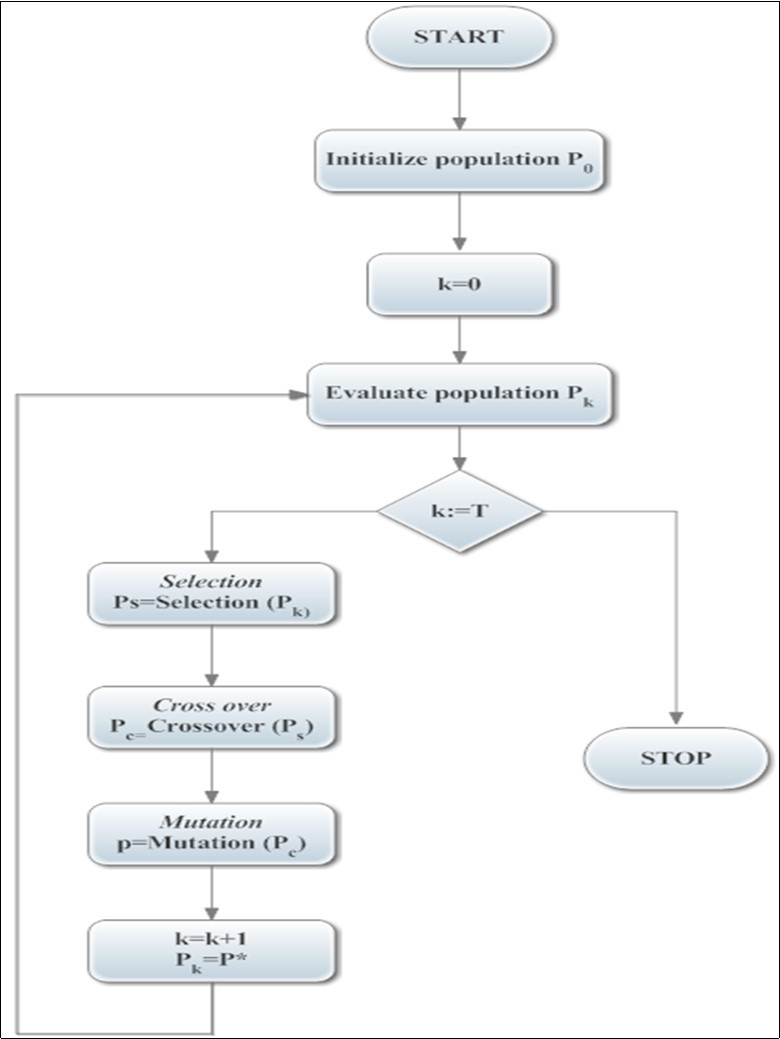1. Reproduction

2. Crossover

3. Mutation

Reproduction is a process in which individual strings are copied according to their objective function values. The operator, of course, is an artificial version of natural selection, a Darwinian survival of the fittest among string creatures. In natural populations fitness is determined by a creature’s ability to survive predators, pestilence, and the other obstacles to adulthood and subsequent reproduction. In our case the apparent resistivity data has been chosen. The best fit optimized true resistivity data was the output that better survives in the selection operator. Reproduction process with individual strings is on the basis of apparent resistivity data. The dataset provides the necessary population generation needed for the natural selection of the algorithm.

Genetic Algorithm (GA) description:

step1: Generation = 0;

step2: Initialize M (Generation);

Evaluate M (Generation);

step3: While (GA has not converged or terminated)

Generation = Generation + 1;

Select M (Generation) from M (Generation - 1);

Crossover M (Generation);

Mutate M (Generation);

Evaluate M (Generation);

End (while)

step4: Terminate the GA.

Hybrid Genetic Algorithm Optimization on Neural Networks

Many optimization algorithms can be used to interpret the Vertical Electrical Sounding data. But most of the optimization algorithm doesn't behave in the way to represent the exact lithological features. But when the optimization algorithm concepts are integrated, the performance of the architecture has been improved. One of such method is integrating the GA and ANN. Many works based on Geoelectrical resistivity data has been going on to converge the interpreted results to a unique solution. Earlier in 1930’s many researchers investigated the inverse problem 21, 22, 23, 24. Later the improvement of computational facilities in 1980s there has been considerable improvement in the data collection and interpretation. The interpretation of vertical electric sounding data was greatly marked by three events: (i) the linear filter theory 25, (ii) the widespread use of digital computers, and (iii) the application of general linear inverse theory. With the latter, the concept of automatic inversion and analysis became popular, in which computer programs resulting from this theory were able to find the best-fitting model automatically, provided they were given a reasonably accurate initial model or a priori information 25, 26, 27, 28, 29, 30, 33.

Since the last decades the optimization techniques, especially global optimization techniques, are widely used for the inversion of resistivity data and other geophysical related problems.

Sen et al. in 1993 3 introduced the application of nonlinear global optimization method namely simulated annealing to the direct interpretation of resistivity sounding data. Chunduru et al, in 1995 31 applied three non-linear algorithms metropolis simulated annealing, very fast simulated annealing and a genetic algorithm (GA) to invert synthetic resistivity data for the classical models embedded in a uniform matrix of contrasting resistivity. The GA technique has also been applied for solving the layer parameter estimation and ground water problems 32.(Figure 4, Figure 5, Figure 6 and Figure 7) shows the VES interpretation for different stations in Rajapalayam-Alangulam belt. Stanley Raj, et al. 34, 35 proved the multiplayer model for evaluating the subsurface parameters easily. Thus regression of multilayer model will provide the exact layer parameters of the earth. Table 1 shows the comparison of conventional method to the GA-ANN model output which proves that the minor subsurface layers also can be extracted using this algorithm. If there is a small deviation in the curve of VES will produce a discriminate change in the layer parameter output. (Figure 8) shows the genetic algorithm population and fitness for Neural networks optimization function. (Figure 9, Figure 10, Figure 11 and Figure 12) shows the 3d plot for genetic algorithm optimizing ANN function and the performance in terms of reduced error percent is depicted.

Table 1. Comparison of conventional and GA-ANN optimization results on geoelectrical resistivity data
 Stations Location Conventional Method Genetically optimized ANN True Resistivity Depth True Resistivity Depth Station 1 Longitude 9.39494 68 1.5 27.9 2 Latitude 77.611 53.8 22.4 44.46 10 936 48.35 25 71.59 30 82.42 50 405.5 60 120.8 80 148.36 Station 2 Latitude 77.6463 20.9 1.24 4.7 2 Longitude 9.37541 40.3 9.29 30.33 10.5 218 37.09 30 105.83 Station 3 Longitude 9.36231 15 4.53 132.47 2 Latitude 77.6926 49.4 14.4 86.21 15 253 85.71 30 96.16 45 106.76 50 128.1 80 168.5 Station 4 Longitude 9.47087 32.5 1.61 32.8 2 Latitude 77.6269 57.9 21.8 65 25 426 366

Figure 4.Genetically optimized Neural Networks geoelectrical inverted layer model for Station1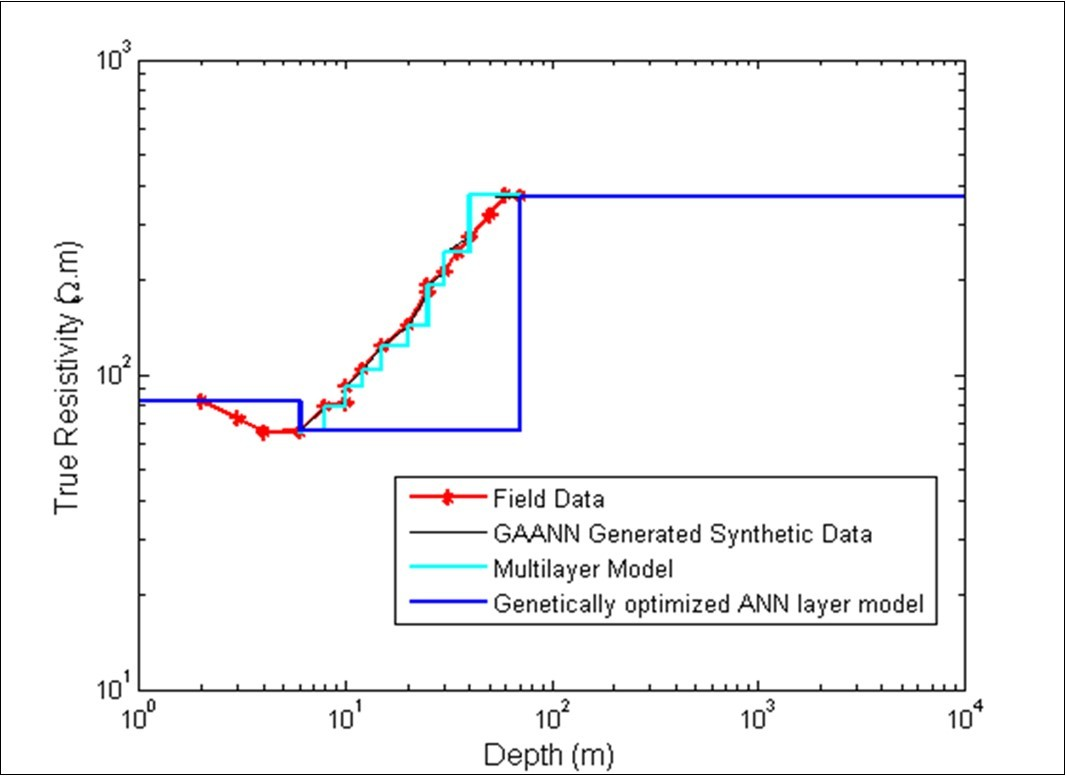Figure 5.Genetically optimized Neural Networks geoelectrical inverted layer model for Station 2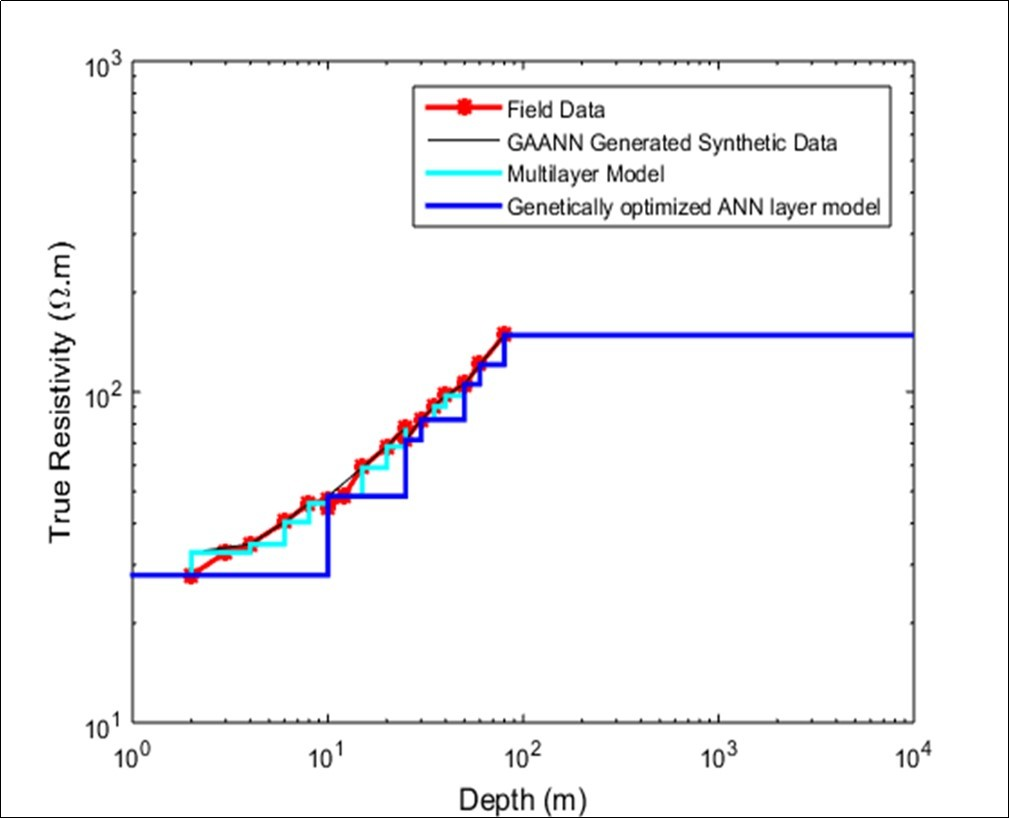Figure 6.Genetically optimized Neural Networks geoelectrical inverted layer model Station 3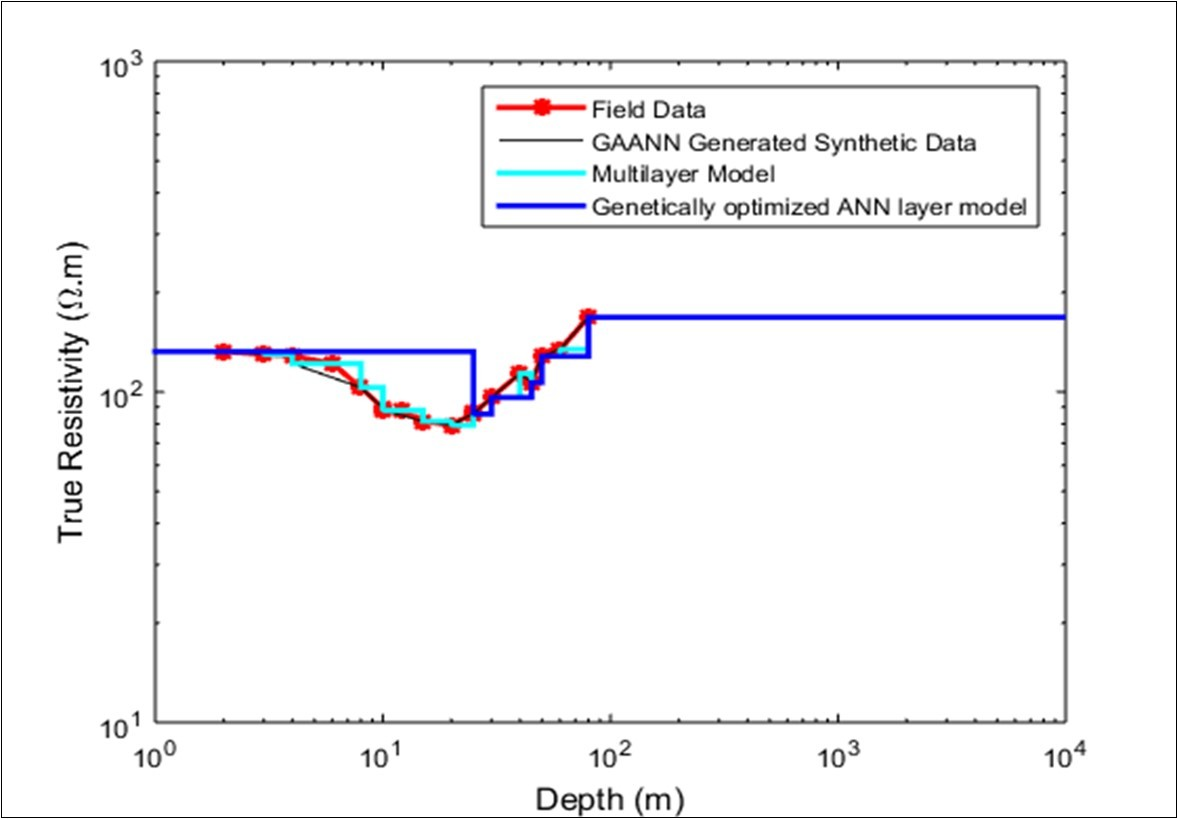Figure 7.Genetically optimized Neural Networks geoelectrical inverted layer model Station 4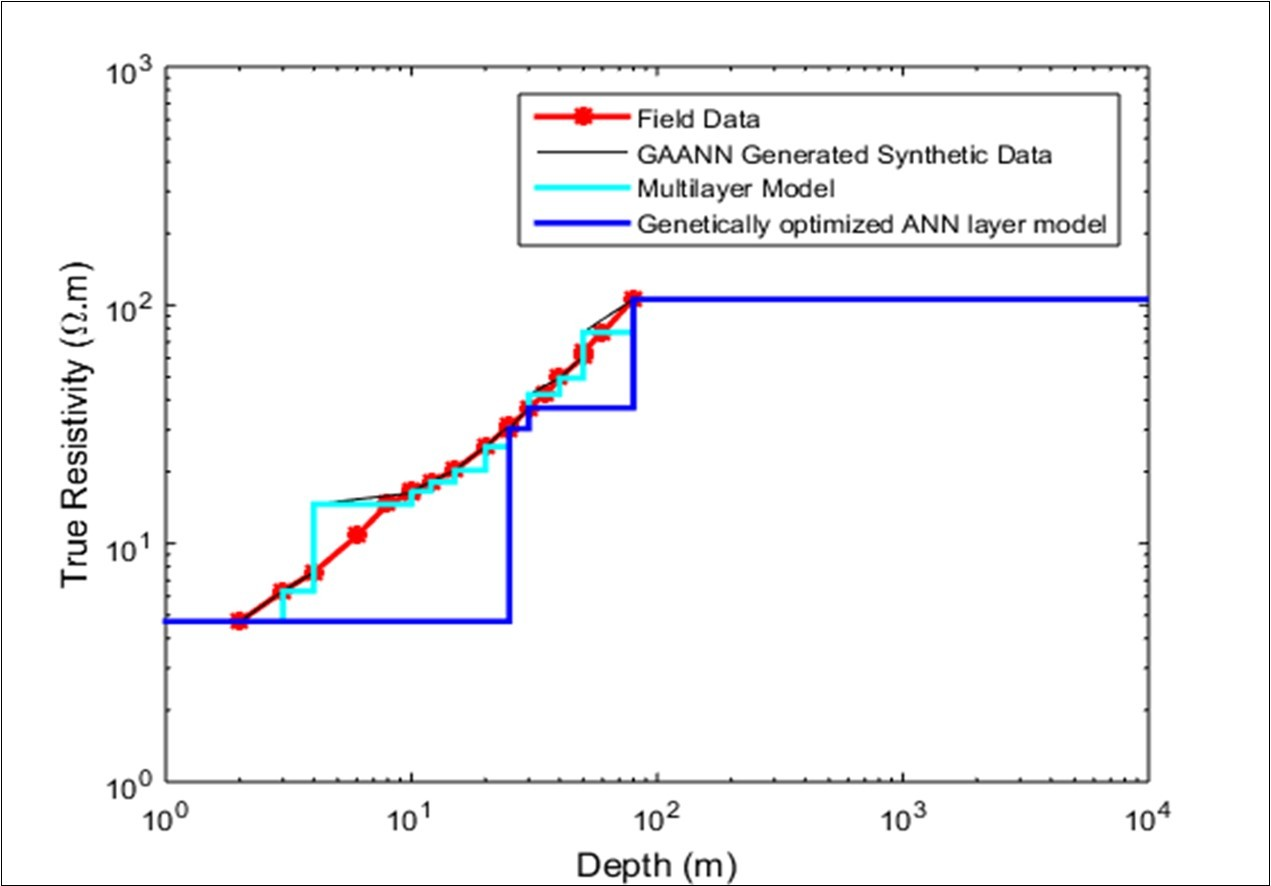Figure 8.Graph showing the Genetic algorithm best estimates and fitness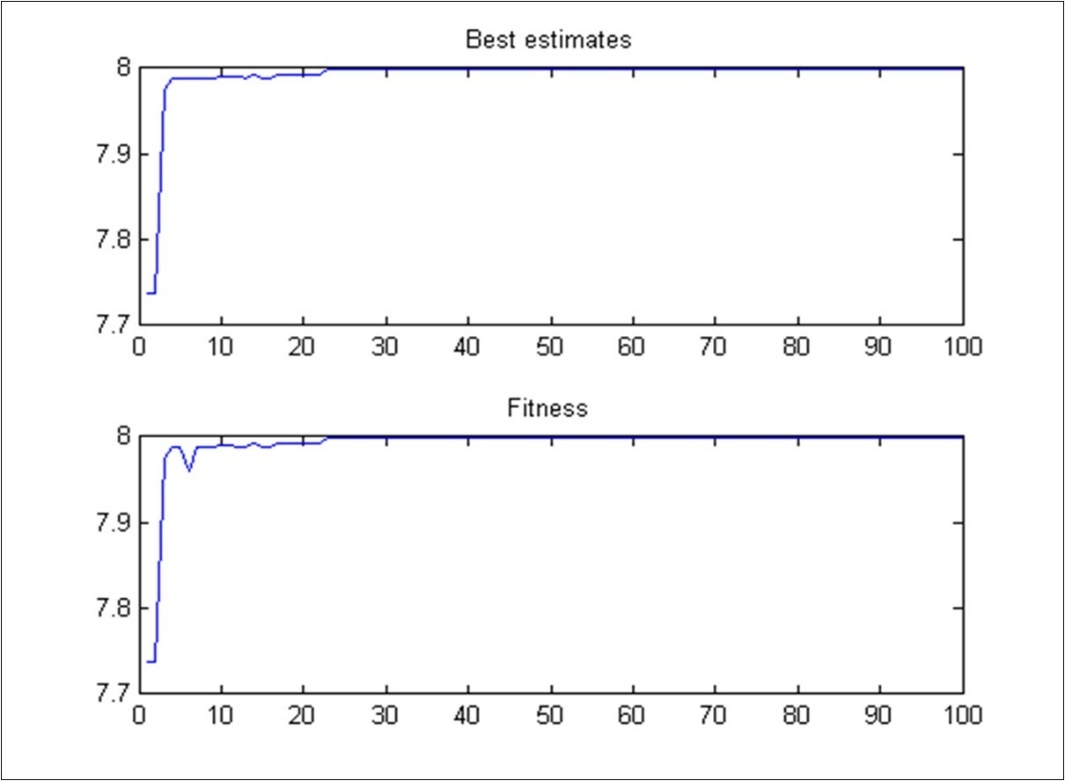Figure 9.Three-dimensional model for population size, fitness and error percent of GA-ANN for geoelectrical resistivity inversion for station 1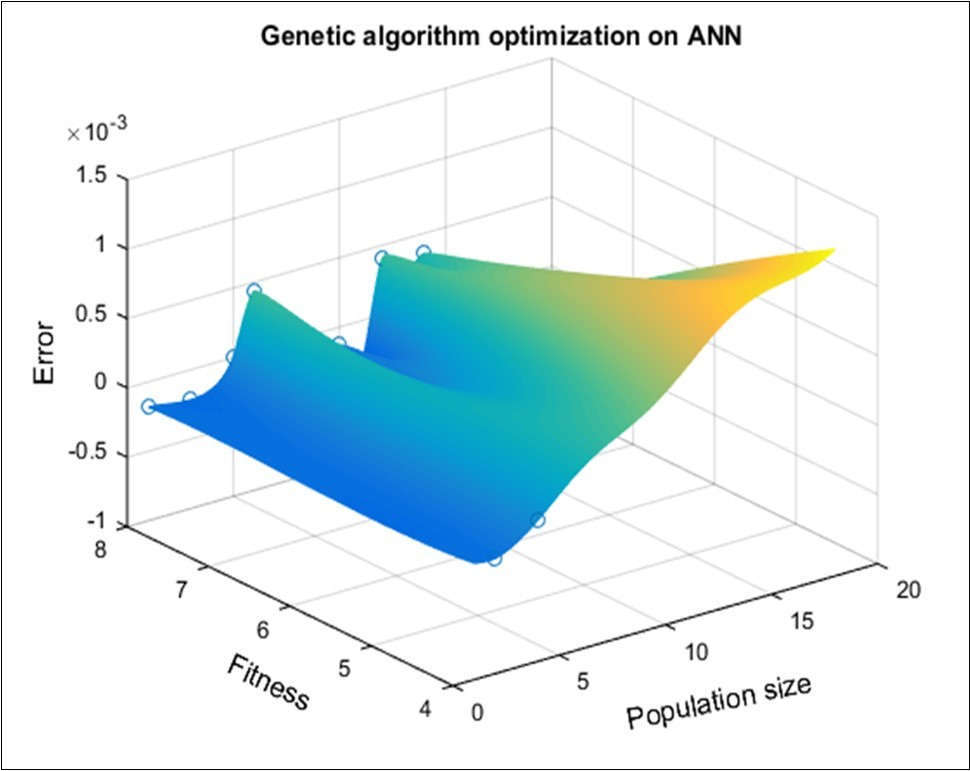Figure 10.Three-dimensional model for population size, fitness and error percent of GA-ANN for geoelectrical resistivity inversion for station 2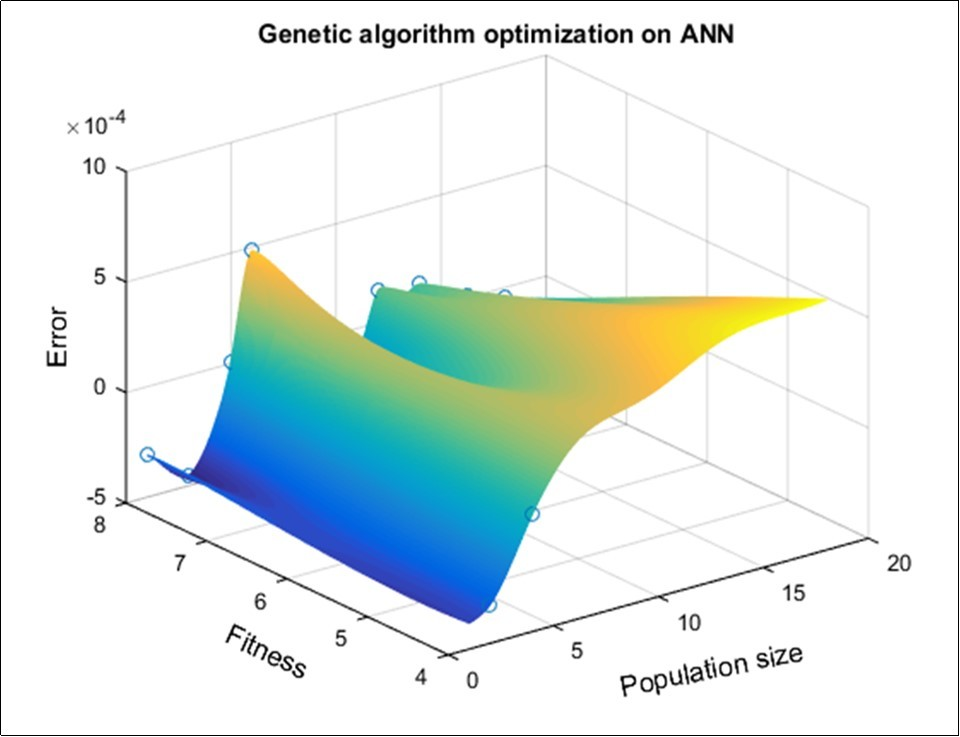Figure 11.Three-dimensional model for population size, fitness and error percent of GA-ANN for geoelectrical resistivity inversion for station 3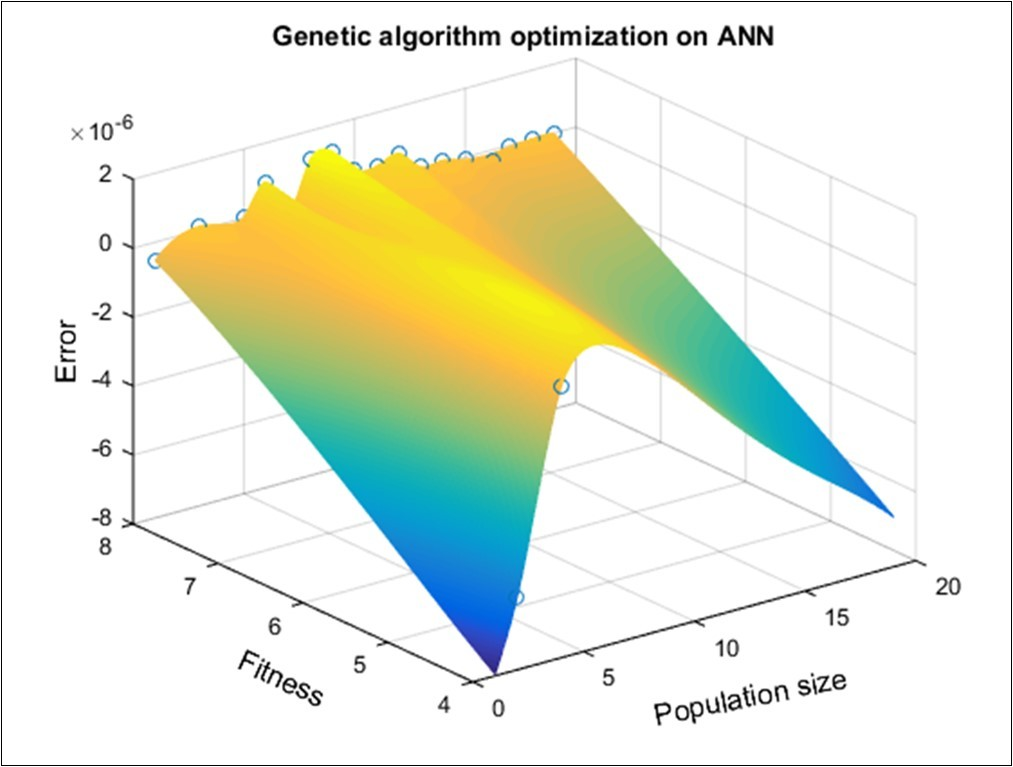Figure 12.Three-dimensional model for population size, fitness and error percent of GA-ANN for geoelectrical resistivity inversion for station 4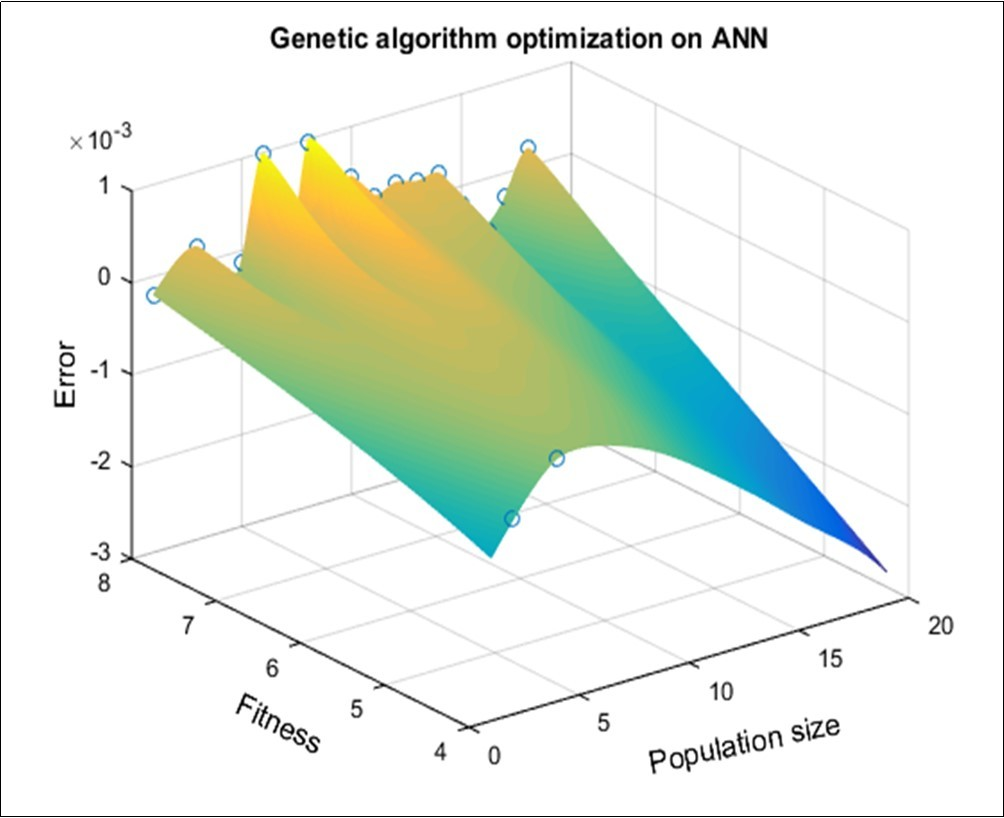## Results and Discussion

For the field data validation, VES data collected from Rajapalayam-Alangulam belt has been chosen for evaluating the performance of GA coupled ANN algorithm. Table 1 shows the interpretation of VES data using conventional and GA-ANN method. It reveals that using GA-ANN technique we can extract very minor layer information of earth’s subsurface and it is proved to be efficient. If the geology is more complex, conventional algorithm cannot be expected to have better performance and we need to apply such artificial intelligent techniques.

## Conclusion

Thus, on summarizing hybrid GA-ANN model always surpasses other algorithms, especially the converging technique. The predicted accuracy of GA-ANN expresses best performance result of more than 90%. Finally, the output layer parameters were modeled. In this model, it converges more quickly and reduces the complexity involves in the non-linear problem. Specialized behavior of GA-ANN algorithm proved that this can be applied to complex geological data and the results are satisfactory and also it can be employed to all kinds of non-linear geophysical inversion problems.

GA-ANN coupled algorithm addresses the geoelectrical data inversion using hybrid approach. In this study, optimization of neural networks is done by genetic algorithm and the local convergence is made using multi node neural networks. On comparing with the traditional approach, this hybrid algorithm has a suitability to predict the subsurface layer parameters effectively. Errors and noises are easily removed through this hybrid approach because of the optimization of objective function of neural networks is made by genetic algorithm.

## Cited by (1)

1. 1.Raj Stanley, Angelena J.P., Hariharan , Kumar D. Senthil, Akshaya S., et al, 2021, Nature Inspired Bargain Optimization Algorithm for Effective Interpretation of Geoelectrical Data, Journal of Current Scientific Research, 1(2), 24, 10.14302/issn.2766-8681.jcsr-21-3796